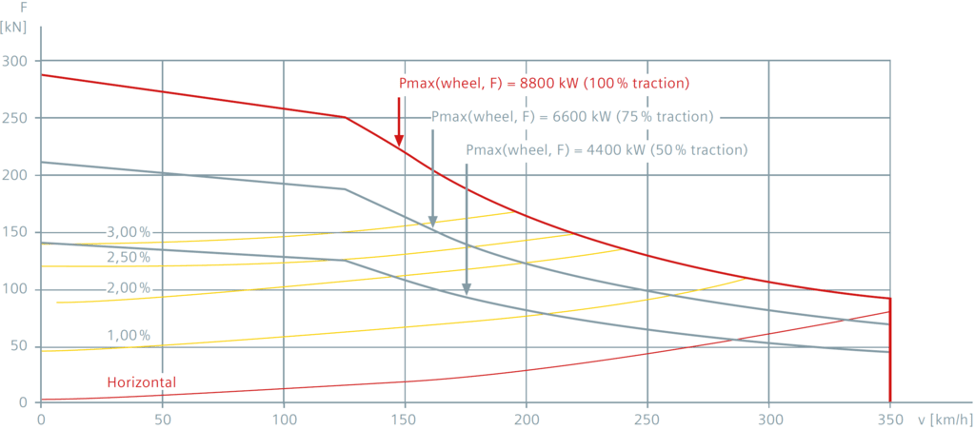In our introductory article, we provided an overview of our approach to developing an energy strategy for the California High Speed Rail System (HSR). In part two of this three part series, we will explore how TerraVerde applied physics to understand the power needs of HSR’s proposed trains.

TRAIN PHYSICS

In many cases, energy procurement strategies begin with a detailed evaluations of historical usage. In some cases, potential load growth considerations (i.e. electrification of systems or fleets, operational expansion) must be evaluating and incorporated into the modeling of expected future energy usage. In the case of HSR, this evaluation required 100% of the energy usage data to be simulated, as the system is not yet operational.

As there were no existing models available to reference for the HSR operation, TerraVerde had to model each mile of the track and calculate the power draw (energy) required to propel the train. TerraVerde applied physics to simulate electricity usage patterns. We referenced the geography and schedule of the proposed route, and used performance data from a proxy trainset with similar characteristics.

The Siemens Velaro E is a good approximation of the trains that will be used in the HSR system. Detailed performance data for these trains is not publicly available. However, we were able gather enough reference points to develop a sufficient model. This tractive effort diagram shows how acceleration, deceleration, and power requirements are related to speed and track slope.

Velaro Tractive Effort DiagramBuilding HSR’s energy use model began with Newton’s 2nd Law:

a = F / m

Where:

• a = acceleration of the train (m ⁄ s^2)
• F = sum of the forces acting on the train (N) = force of the motor – resistance forces = tractive force – drag, friction, rolling resistance
• m = mass of the train (kg) = 439,000 kg + mass of the passengers

The power output capabilities  of the trains’ electric motors are known. The key to calibrating our model was quantifying the resistance forces. Drag (i.e. air resistance) played a significant part in the HSR model, because drag is proportional to the square of velocity. At top speed, the total resistance force is approximately 8 metric tons on flat terrain.

TerraVerde then used a polynomial to represent how resistance forces change relative to speed and incline:

F_resistance = mgSIN(θ) + a + bv + cv^2

Where

• θ = angle of incline of the tracks
• g = gravitational constant = 9.81 m ⁄ s^2
• v = velocity of the train (m ⁄ s)
• a, b, and c are constants, these values determine the shape of the resistance curves

In our model, we solved for values a, b, and c to calibrate our resistance curves to match those reflected in the Velaro’s tractive effort diagram.

This formula became the basis from which we were able to determine train performance and power draw as it traveled the proposed routes, as well as how much power it generated as it applied brakes. From this model, we were then able to simulate a year’s worth of energy usage, and begin calculating projected electricity billing rates for HSR.

Coming up next, in our final installment in this three-part blog series, we will cover how TerraVerde used this power draw model to evaluate an optimal energy procurement strategy. We’ll discuss wholesale vs. retail market options, solar & battery energy storage options, and resiliency (backup power) considerations.

TerraVerde Energy provides a wide range of independent advisory services to support CCAs, public agencies, and commercial enterprises with evaluating, deploying, and managing smart energy programs. To learn more, write to us at hello@terraverde.energy.

Stay in the know with our latest blog posts!

By submitting this form, you are consenting to receive marketing emails from: TerraVerde, 1300 22nd Street, San Francisco, CA, 94107, http://terraverde.energy. You can revoke your consent to receive emails at any time by using the SafeUnsubscribe® link, found at the bottom of every email. Emails are serviced by Constant Contact